# Demand forecasting and dynamic pricing

Damavis demand forecasting model and dynamic pricing strategy

## 1. Introduction

Demand forecasting and suitable pricing are two key factors in any business. In the hotel context in particular, and more specifically in the online segment, these tasks are of vital importance due to the dynamic nature of prices and high competitiveness. Automating these processes is key to a quick adaptation, which is why more and more hotels are supporting their decisions in recommendation systems or RMS.

In this article, we present a hotel demand forecasting model and a strategy for choosing the optimal price in order to maximize expected profits. The structure of the article is summarized below.

In section 2 we describe the data set used for this case study. In section 3, we present the demand forecasting model, based on time series.  In section 4, we explain how the above prediction is used to generate possible future scenarios through Monte Carlo simulations. In section 5, we will study the effect of price on demand from historical data. These results will be used in section 6 to calculate the optimal price on the simulations generated in section 4. In section 7 we present the results obtained in each of the previous sections. Finally, in section 8 we summarize the work done and suggest further improvements.

## 2. Data description

The data set we use is a three-year simulation of bookings at a certain hotel, in whose data we have included the effect of weekly and yearly seasonality. We will use the data up to a certain date, the in-sample period, to predict future demand and propose an optimal price over the remaining dates, the forecast period.

It is assumed that the hotel accepts reservations for a specific day of arrival since 𝑇 = 10 days in advance, up until the same day of arrival. The prices are fixed once a day, so at the beginning of each day the hotel manager sets the prices for the current day and the next 𝑇days. These prices are kept during the whole day, so all reservations made on that day are charged these rates. However, the next day, the corresponding 𝑇 + 1 prices are set again, which may be different from those set on the previous day. The hotel capacity is 𝐶 = 100 rooms.

We have detailed information of price and total demand for each day of arrival and each booking day. The dataset also provides the period or season in which each check-in day is included, both for historical data and for the dates to be predicted. In this case there are four distinct periods:

• Very Low Season (Very Low): December, January and February.
• Low season (Low): March, October and November.
• High season (High): April, May and September.
• Very High Season (Very High): June, July and August.

Thus, the data set has the following columns:

1. ReservationDate: the day on which the reservation is made.
2. CheckInDate: the day of check-in.
3. ADR: the price set on the day of reservation for that day of check-in, in dollars.
4. Demand: number of reservations on the reservation date for the check-in date.
5. Season: the period in which the check-in day is included.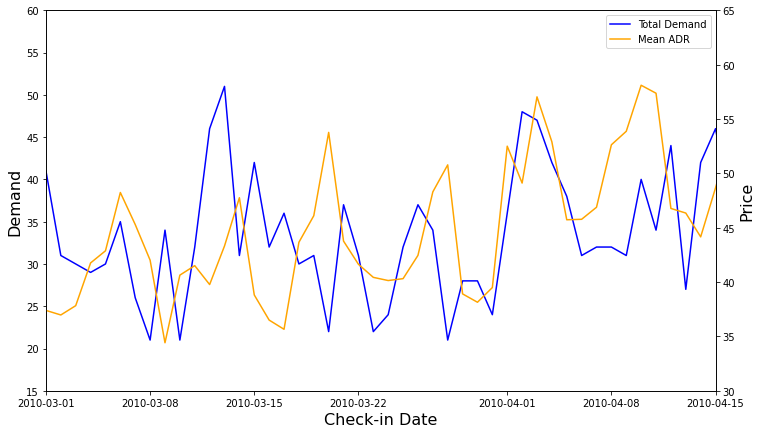Figure 1. Above, evolution of total demand and average price for each check-in day in our dataset, in which the annual seasonality and the positive correlation between demand and price can be observed. Below, detail of the previous graph, where the weekly seasonality can be appreciated.

Figure 1 shows both aforementioned annual and weekly seasonality, as well as a slight upward trend in both demand and price over time. A strong positive correlation between price and demand is also observed, as is often the case in the hotel industry.

## 3. Prediction of future bookings

We now define the process by which we predict future demand. Let 𝑅(𝑖, 𝑡) be the total number of reservations made for a particular check-in day 𝑡, a number of days 𝑖 in advance (difference in days between booking date and check-in date). Note that this number is a realization of the bookings random variable, which we denote by 𝐵(𝑖, 𝑡). We model this random variable as the product of two independent functions as follows.

On the one hand, the size of demand according to arrival day, 𝑠(𝑡), represents the total number of expected reservations for a 𝑡 check-in day. On the other hand, the demand shape as a function of advance, 𝐵'(𝑖), represents the fraction of the total number of reservations for a particular arrival day that are made 𝑖 days in advance.

### 3.1. Demand shape

We assume that the demand shape as a function of the booking advance time depends on the season (or period) in which the arrival day 𝑡 is included. As we mentioned in Section 2, there are four seasons in our data, corresponding to periods of very low, low, high or very high activity. Then, for each season 𝑘, we consider the set 𝑆𝑘 of all days falling into that period, and we can estimate the form of demand as a function of anticipation as follows.

That is, for each arrival day of the season under consideration, the fraction of reservations made 𝑖 days in advance is calculated. Finally,the average over all days is computed in order to obtain the demand shape average for period 𝑘. It is assumed that this pattern does not vary in the short term, so the calculated seasonal demand shape will be used in the estimation of future demand.

### 3.2. Demand size

Demand size represents the total expected demand for each check-in day. It is thus estimated from the sample data as the total number of reservations made for each particular stay date.

Remember that our data presents both annual and weekly seasonality. In order to predict the series behavior and thus be able to estimate demand in the future, we deseasonalize it as follows.

First, we calculate the seasonal index for each season 𝑘, using to the formula

where the denominator represents the average of the series 𝑠(𝑡) in the year which day 𝑡 belongs to. Thus, seasonal index for period 𝑘 is just the average demand for this period, where each value is normalized by its annual average. Then, let 𝑘𝑡 be the period to which day 𝑡 belongs, we calculate the yearly deseasonalized series, as follows

where the subindex 𝑌 represents that this is an intermediate step where yearly seasonality has been removed.

To get rid of weekly seasonality, we follow a similar process. This time, the series 𝑠𝑑𝑒𝑠,𝑌(𝑡) is considered and we use the day of the week 𝑑 instead of the period 𝑘. We also use the median instead of the average to represent the seasonal index of each day of the week. This technique is common in the literature in order to preserve the shape of the weekly peaks. Therefore, we will calculate the seasonal index for day of the week 𝑑 as

where 𝐷𝑑 represents the set of all days that are of type 𝑑 (e.g. all Mondays). Here, the denominator represents the average of the series  𝑠𝑑𝑒𝑠,𝑌(𝑡) over the week containing day 𝑡. Finally, the deseasonalized series is computed as

where 𝑑𝑡 represents the day of the week of each day 𝑡.

Once we have stripped seasonality out of the series, we forecast it using the Holt-Winters exponential smoothing model, whose parameters are estimated from the sample data. Another option is to use SARIMA models, which require finding the hyperparameters (p, d, q, P, D, Q, m) that best fit the available data. In our case, since we have already deseasonalized the series, we have not found great improvements in the results when using these models. For this reason and due to its relative computational complexity, we use the exponential smoothing approach.

After making the projection of the deseasonalized series, we must undo the previous transformations in order to obtain the total estimated demand for each arrival day. This means that for each day 𝑡 of the forecast period, we must multiply the value of 𝑠𝑑𝑒𝑠,𝑌(𝑡) by their respective seasonal indices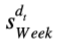and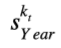, where 𝑑𝑡 and 𝑘𝑡 are, respectively, the day of the week and the season corresponding to day 𝑡. In this way, we obtain the estimate for the future demand size.  The results are shown in section 7.

## 4. Demand simulation

Next, we simulate the expected future demand projection assuming that the pricing policy will not change. Thus, the simulated demand is a reference value that will be affected by the pricing policy that is decided to be followed.

We modeled demand as a product of two independent functions, the demand size and the demand shape. For each day in the period to be predicted, we have predicted the demand size ŝ(𝑡) by projecting the deseasonalized series ŝ𝑑𝑒𝑠(𝑡), subsequently multiplying the value by the corresponding seasonality indices. On the other hand, we have obtained an estimate of the form of demand Ḃ𝑘(𝑡) for each period 𝑘 from the historical data. Thus, the expected number of bookings made for a certain arrival day 𝑡 with an advance of 𝑖 days is computed by means of the product

where 𝑘𝑡 is the period in which day 𝑡 is included. In order to simulate the data, we also need to know the variance of the random variable 𝐵(𝑖,𝑡). Since we cannot calculate the variance explicitly, we approximate it from in-sample data as the mean squared error of the bookings variable for all days belonging to the same category, i.e., the same day of the week and the same period, and the same advance 𝑖. Thus, we compute the variance of the booking variable as

where 𝑆𝑘𝑡 is the set of days belonging to the same season 𝑘𝑡  as day 𝑡 and 𝐷𝑑𝑡 the set of days of the same day of the week 𝑑𝑡 as day 𝑡.

From these statistics, we simulate the forward demand for each pair (𝑖, 𝑡) as a normal distribution with average B(𝑖,𝑡) and variance Var( B(𝑖,𝑡)). This distribution is left-truncated at zero, since it is not possible to have negative demand values. Note that the normality assumption could be tested and, if rejected, a different model could be proposed. However, here we have considered enough to control for the average and the degree of dispersion of the demand.

Next, using the Monte Carlo method, we simulate 𝑀 possible demand trajectories for the forecast period. Thus, for each day 𝑡 and each lead time 𝑖 from 0 to 𝑇 (the maximum lead time), we take 𝑀 values from a normal distribution with mean and variance given by equations (8) and (9) respectively.

## 5. Estimation of the price – demand relationship

In order to find the optimal price to be set in order to optimize profits, one must take into account the effect that price has on demand.  We know that in general the law of demand holds true: a rise in price leads to a fall in demand, and vice versa. However, when studying the data we generally find a positive correlation between price and demand. This is because there are common variables affecting both price and demand, a problem known as price endogeneity. For example, in general we have more potential customers in high season, so hotel managers raise prices, and yet demand is higher than in low season.

In this context, a simple estimate predicts a positive elasticity value, i.e., that a rise in price causes a rise in demand, contrary to the law of demand. This is shown in Figure 2.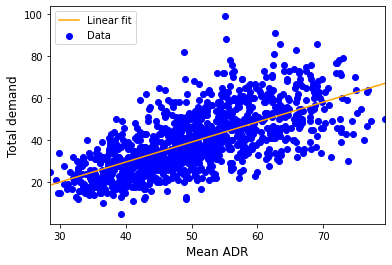Figure 2. Linear regression of total demand on average price per day of arrival 𝑅² = 0.441. We observe that the slope is positive due to the endogeneity of the price variable.

Therefore, we need to process the data in some way to find the real effect of price on demand, which in particular must be negative. To this end, we proceed as follows.

First, we divide the data into similar subsets, that is, we group together days that belong to the same season and are of the same day of the week. For each subset, we calculate the average of the prices and the number of reservations obtained, which will be the reference prices and demands for that subset. Finally, we normalize the historical prices and demands by dividing each of the prices and demands by its corresponding reference value.

In this way, we hope to isolate the causal effect of varying price on demand. For example, a normalized price greater than 1 means that the price set was higher than the average price under the same conditions (e.g. because the hotel was almost full). Such a choice might result in a lower demand than the average demand under the same conditions, so the normalized demand would be less than 1.

In this case, we consider a linear regression of normalized demand on normalized price for each of the 4 seasonality periods. After fitting each model using the data for each period, we have that

where 𝑝𝑛𝑜𝑟𝑚(𝑖,𝑡) and 𝑑𝑛𝑜𝑟𝑚(𝑖,𝑡) are the normalized price and demand for a certain arrival day 𝑡 and lead time 𝑖, and 𝑘𝑡 is the period to which day 𝑡 belongs.

In the next section, we use this relationship to predict how expected demand will increase or decrease depending on the price we set on each particular day. In this way we can calculate the expected profits for a particular price choice more reliably.

## 6. Optimal price calculation

In this section we define the strategy followed to find the optimal price. The objective is to fix the set of prices that maximizes profits in the forecast period, which implies a choice of price for each day 𝑡 and each possible lead time 𝑖.

If we do not impose any restrictions on the behavior that the price should follow, this problem can become extremely complex. In particular, the choice of the optimal price given a certain time in advance 𝑖 depends on the expected profits to be obtained in the forthcoming days, which in turn depend on the price to be set for each of these days. For example, if the hotel is not expected to fill up, the price to be set should be relatively low to ensure a certain occupancy, while the price may be higher if high demand is expected. Such an approach requires solving a recursive problem, for example using dynamic programming.

As we will see in a future post, this calculation can be relatively complex even in simplified situations.  To simplify this calculation, we present a parametric model for price based on multipliers.

### 6.1. Dynamic pricing model using multipliers

Let us assume the following approach. The price to be set on each day 𝑡 and each advance 𝑖 could depend on several factors, each of which contributes to setting the final price. In particular, these factors, centered at 1, are interpreted as discounts or premiums that will be multiplied to a reference price. Here, the main assumption of the model lies in the fact that the multipliers are linear with respect to their respective variables, as we will see below. In particular, we take into account two factors: the number of rooms available and the number of days in advance of booking.

#### 6.1.1. Availability multiplier

A common practice in the hotel industry is to increase the price as the number of rooms available for the day in question decreases. Thus, in our model the multiplier decreases linearly with the number of rooms available, as seen in Figure 3.

The multiplier is determined by two parameters. The minimum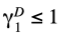is given when the hotel is empty and the maximum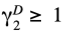when the hotel is full.

It is common that the room price for a particular day increases over time since the moment reservations are accepted, and that a discount occurs in the last days if rooms are still available. Thus, the multiplier starts from an initial value of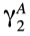,  at the maximum advance of  𝑇 days,  and increases linearly up to a maximum of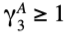at a certain advance time of 𝑡1  days. From this point, it decreases linearly to a minimum of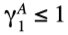on the same day of arrival. The parameters of the advance multiplier are thus,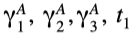.

#### 6.1.3. Restrictions on parameters

Let us note that the two multipliers that have been defined depend on a set of 6 parameters (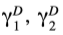for the availability multiplier,for the advance multiplier). The form of the multipliers imposes the following constraints on these parameters so that they produce logical results:

Thus, the choice of the optimal price will consist of finding the set of parameters that maximizes profits in the forecast period. To reduce the number of parameters to be determined, we impose additional constraints on the parameters. The purpose of the multipliers, as explained, is to introduce variations from a reference price, so we impose the average of the multipliers over the range of possible values to be 1. Thus, the following additional constraints are obtained:

In this way, the set of parameters to be fixed is reduced to (). The remaining two parameters are determined from these by equations (14) and (15).

### 6.2. Optimization process

#### 6.2.1. The objective function

The first step in any optimization problem is to define the objective function to be optimized. In this case, what we want is to maximize total profits in the forecast period. It should be noted that this model could be adapted to different goals depending on the customer’s needs. For example, one might prefer to maximize occupancy instead of benefit, even adding the constraint of ensuring a certain profit.

The above multiplier model allows us to reduce the search space to the four aforementioned parameters (). Thus, the price optimization problem is reduced to finding the best combination of these parameters. Next, let’s see how we calculate the expected profits given a set of parameters, using the generated Monte Carlo simulations and the effect of price on demand.

Note that, given a combination of parameters, for each arrival day 𝑡  and number of days in advance 𝑖, the value of the multipliers will be different. This is because each multiplier depends on a state variable, in particular on the number of rooms available 𝑎(𝑖,𝑡) for day 𝑡  when we are in 𝑖 days in advance, and on the advance 𝑖 itself. Thus, the price set for each pair (𝑖,𝑡)  is calculated as

where the subscripts 𝐴 and 𝐷 denote the advance and availability multipliers respectively, and the values 𝑝𝑟𝑒𝑓(𝑖,𝑡) represent the reference price. This reference price can be set by the hotel manager himself. However, in this case we take it as the seasonal average obtained from historical data.

To calculate the effect of price on demand, we must take into account the relationship found in section 5. Recall that the normalized price is obtained by dividing each price by its reference price. Thus, according to equation (16), in this case normalized price is just the product of the multipliers. Using equation (10) we can then estimate the normalized demand, which represents the variation with respect to the reference demand caused by the price. Therefore, the number of expected bookings for those values of (𝑖,𝑡) should increase or decrease depending on whether the normalized demand is greater or less than 1, respectively. We do this as follows:

• If the normalized demand is higher than 1, i.e. 1 + 𝑥, each existing reserve is kept  and a duplicate is created with probability 𝑥.
• If the normalized demand is less than 1, say 𝑥, each existing reserve is maintained with probability 𝑥.

Thus, given a set of parameters (), we will loop over each Monte Carlo simulation as follows:

1. The profit is initialized to zero.
2. For each day of arrival 𝑡 and each day of reservation 𝑖 days in advance:
• The price set is calculated according to the value of the multipliers.
• The normalized demand associated with the normalized price is calculated.
• The new demand is calculated according to the procedure explained above.
• The realized demand is calculated: the minimum between the new demand and the number of rooms available. The number of available rooms is updated.

After this process, the total expected profit for each Monte Carlo simulation is obtained. By averaging over all of them, we obtain the expected value of the benefit using the set of parameters ().

Note that, in this setting, the objective function is a stochastic function, since different objective values can be obtained in successive runs of the function, taking as input the same set of parameters. For this reason, it is important that the number of Monte Carlo simulations be large enough to minimize spurious effects when averaging the results.

#### 6.2.2. Optimization strategy

Once the objective function has been defined, the optimization strategy to be followed must be determined. In case of differentiable functions, such as loss functions used in machine learning problems, it is common to use methods based on the calculation of the derivative such as gradient descent. However, as we have seen, in our case the objective function is relatively complex and in particular it is not deterministic. This makes the calculation of its derivative unfeasible, so we must take a different approach

In this case, we use an optimization technique based on an evolutionary algorithm. Such algorithms, in general, follow the general laws of biological evolution (in particular mutation, combined inheritance and natural selection). Thus, starting from an initial number of possible solutions, represented by valid combinations of parameters, the value of the objective function (called the fitness function, which must be minimized) is calculated for all of them. Then, natural selection is simulated by some selection method in which the fittest individuals (this is, the sets of parameters with lower value of the fitness function) are rewarded. Selected individuals breed with each other such that offspring represent a combination of parents’ parameters. In turn, mutations (variations with normal distribution) are introduced in order to explore new sets of parameters. The process is then iterated simulating successive generations, obtaining better and better combinations of parameters.

In particular, for this problem we use the method called CMA-ES (Covariance Matrix Adaptation Evolution Strategy). This model is very flexible, so that it only needs as input the ranges of possible values for each parameter (specified by the constraints of section 6.1.3). Then, the evolutionary strategy is iteratively followed until the value of the objective function is stabilized or a certain fixed number of generations is reached. Finally, the individual with the minimum value of the objective function (here the maximum value of expected total benefits) is chosen as the optimal solution.

Note that this method is not deterministic, even if the objective function was. Due to the intrinsic randomness of the evolutionary strategy, different runs of the algorithm lead to different results, even with the same initial population. Therefore, it is not guaranteed to find the best combination of parameters, but it is suitable for exploring continuous state spaces, as is our case. Thus, we can run the algorithm several times to test with different initial populations, and finally we choose with the combination of parameters that gives the highest total benefit.

## 7. Results

In this section we present the main results obtained in this work. With the purpose of showing how predicted demand preserves annual seasonality, we have used a one-year length forecast period. Specifically, data from 2010 and 2011 are used to train the model in order to predict demand and recommend the optimal pricing strategy for 2012. Data from 2012 is only used to benchmark the obtained results.

In general, the longer the forecast period, the more difficult it will be to achieve realistic results, since there may be factors such as the entry of new competitors into the market or social changes (economic improvements, health crises) that affect demand in the short and medium term. In this case, since the data are simulated, the results are acceptable even for long-term projections. However, for the reasons given above, it will generally only be safe to forecast a few weeks beyond the last day for which data are available.

### 7.1. Demand projection

As explained in section 2, we have considered the time series consisting of total demand for each check-in day of the in-sample period and studied its future projection. To do so, we have adjusted the series’ both annual and weekly seasonality, and predicted its trend using the Holt-Winters exponential smoothing method. The result of this projection is shown in Figure 5.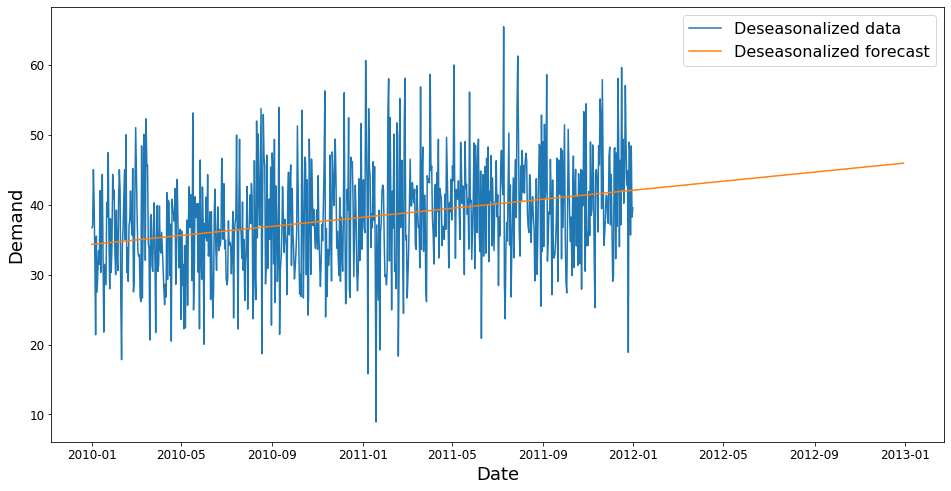Figure 5. In blue, the deseasonalized demand for the in-sample period. In orange, the linear forward projection of the series using the Holt-Winters model.

Finally, after undoing the previous transformations, we obtain the total predicted demand for the forecast period. As we can see in Figure 6, in general the prediction is able to capture the annual seasonality, although in the months from March to June the actual demand is somewhat lower. On the other hand, it is generally observed that the predicted series presents weekly peaks that mainly coincide with those of the real series. However, in general the latter exhibits larger fluctuations that the model is not able to adjust.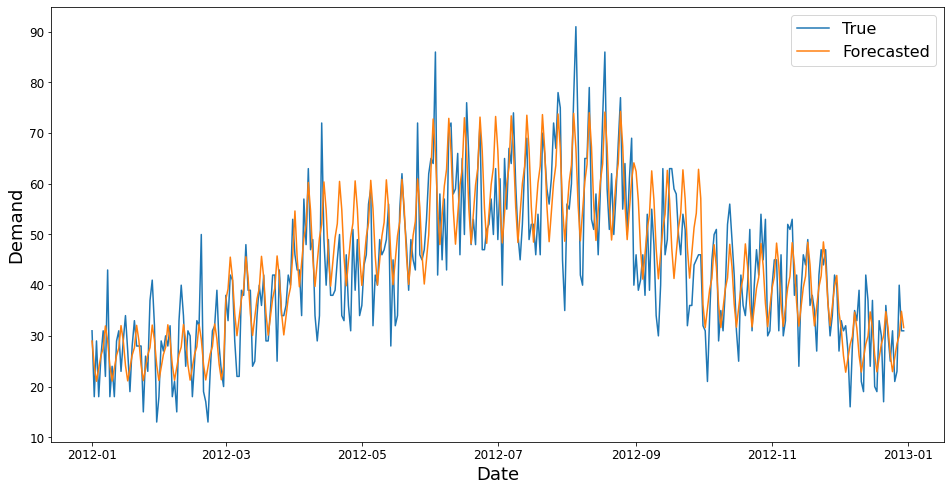Figure 6. Prediction and actual value of total demand in the forecast period, represented in orange and blue colors, respectively.

### 7.2. Form of the demand according to the time in advance

Figure 7 shows the fraction of total reservations made at each advance time for each season considered in the data. In this case we assume that reservations are allowed from 𝑇 = 10 days before the day of arrival.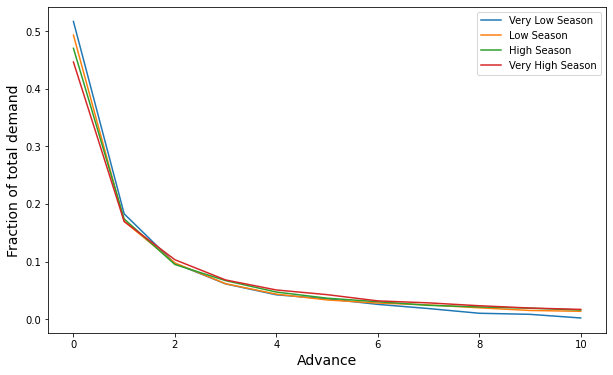Figure 7. Shape of demand for each season calculated according to Equation (2). On the X-axis, the days in advance of the check-in day, and on the Y-axis the fraction of the total demand made on that day on average.

In general, demand is concentrated on the days closest to the day of check-in. This tendency is accentuated in the lower seasons, when more than 50% of reservations are made on the same day of check-in. On the other hand, in the higher seasons, demand is distributed somewhat more evenly throughout the booking period, as clients generally book more in advance.

This demand shape calculated from historical data is considered to not change much in the short term.This, it is used, together with the forecasted demand size, to simulate demand in the forecast period, as shown in section 7.4.

### 7.3. Effect of price on demand

We have studied the effect of price on demand from the in-sample period onwards. As shown in Figure 2, a linear regression of total demand on average price would result in a positive slope, contradicting the law of demand. Therefore, we instead estimated the effect of normalized price on normalized demand, where both quantities were divided by reference values.

A linear regression has been considered for each labelled period in the data, in order to detect different patterns depending on the season. Similarly, different regressions could have been performed for different days in advance or different days of the week. However, due to the limited volume of data, such a detailed analysis could result in an excessive dispersion in the coefficients. As can be seen in Figure 8, after normalization we obtain a negative effect of price on demand in all cases, thus matching the law of demand.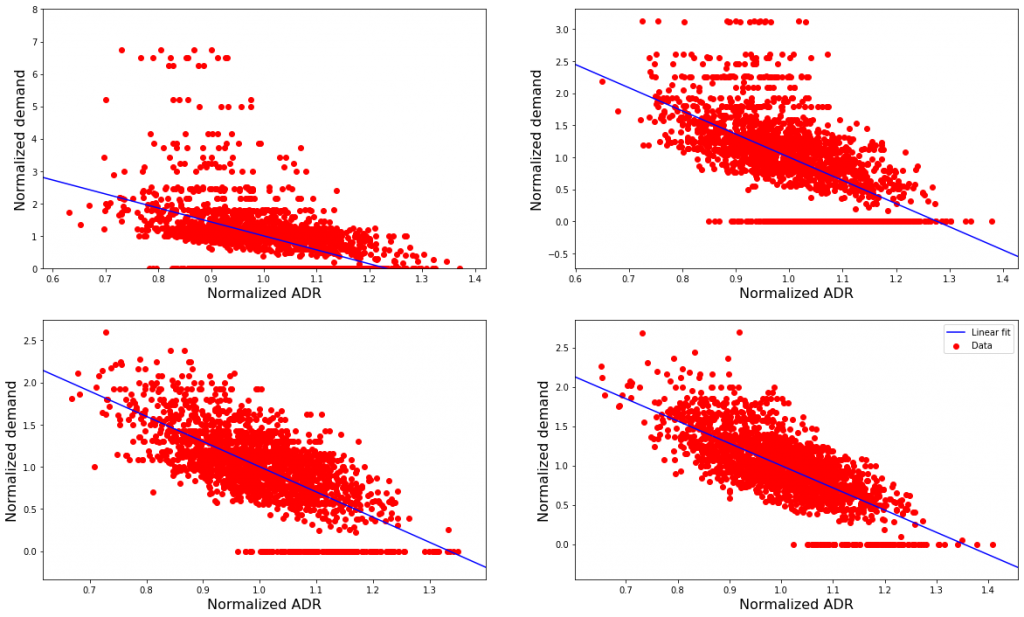Figure 8. Scatter plots of normalized demand as a function of normalized price, together with their linear fit. From left to right and from top to bottom, very low, low, high and very high seasons. The R² values are 0.244, 0.368, 0.468, 0.536, respectively. It is observed that the slope is negative in all cases, with values of -4.315, -3.615, -2.989, -2.833, respectively.

### 7.4. Optimal pricing strategy

First, we performed 𝑀 = 100 Monte Carlo simulations for the demand in the period to be predicted. Each simulation has a demand value for each check-in day (all days of the year 2012) and each possible value of advance (from 0 to 10 days, both included). Figure 9 shows some results of the simulations.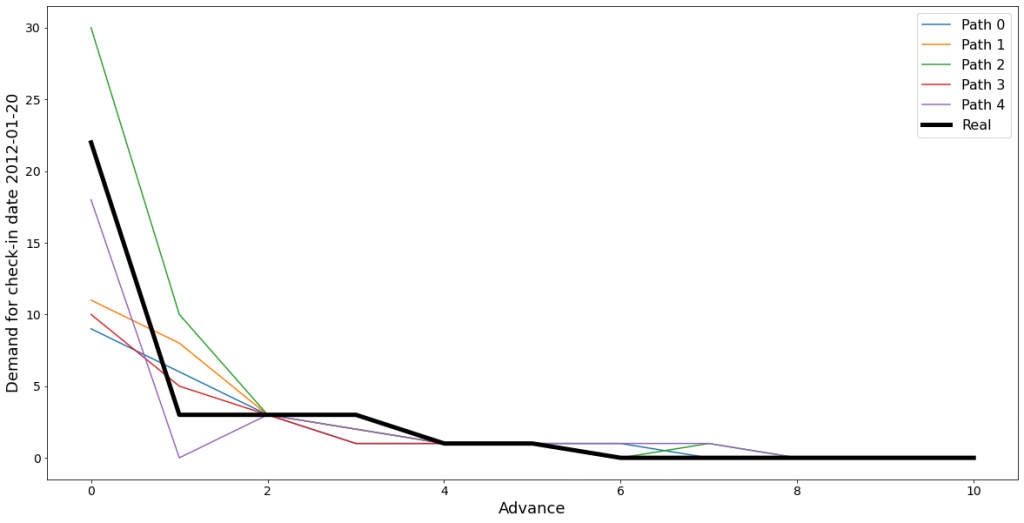Figure 9. Comparison of 5 Monte Carlo simulations with actual data (in black). Top panel displays total demand for each check-in day in the first two months of the forecast period. Bottom panel shows demand as a function of advance on a specific date of arrival (January 20, 2012).

From these simulations, we search for the optimal price using the evolutionary strategy explained in Section 6. In this case, we find that the optimal price is given by the set of parameters () = (1.279, 0.801, 0.979, 0.146). The resulting multipliers are shown in Figure 10.

It can be seen that the recommended price varies considerably depending on the number of rooms available. In fact, the availability multiplier is almost twice as high when the hotel is almost full (few rooms available) as compared to when it is empty (𝐶 = 100 rooms available).

Regarding the advance multiplier, we see it is recommended to keep the price very close to the reference value, slightly increasing its value until one day before check-in. A large discount is offered for the day of arrival in order to obtain a higher last day demand.

In Figure 11, we compare the actual results with the results that would be expected to be obtained with this pricing policy, for a particular check-in day. Note that we assume a demand response in line with that estimated in section 6.2. As an example, July 7, 2012, corresponding to a very high season Saturday, is shown.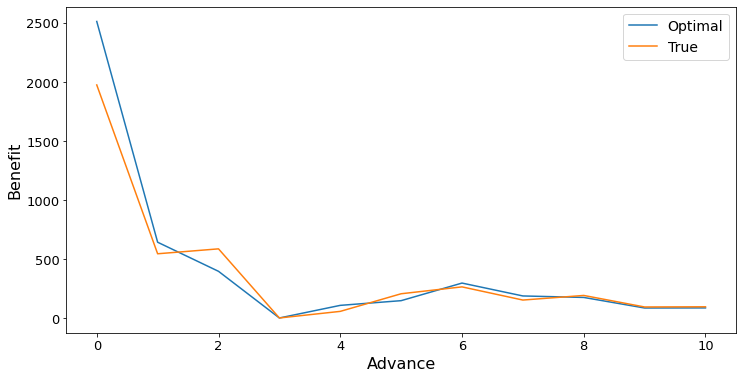Figure 11. From top to bottom, comparison of price, demand and profit obtained on each day in advance corresponding to the July 7, 2012 check-in date. Shown in orange are the actual values and in blue those obtained following the recommended price.

As seen in the top panel, the model recommends a generally lower price than the one currently being charged. This is due to the fact that the reference price has been calculated as a seasonal average from historical data. The dataset presents an increasing trend, so the in-sample period price is lower than that in the forecast period. On the other hand, we observe that a price decrease is recommended on the same day of check-in, according to the advance multiplier. The middle panel shows that this decrease results in a much higher demand on the day of check-in. As can be seen in the lower panel, despite charging a lower price, a higher overall profit is obtained by using the recommended strategy.

The behavior described is similar for all seasons and days of the week: the recommended price is lower than the current price. Intuitively, the increase in demand is expected to compensate for the lower price and thus the expected total profit than the actual one. It must be noted that our dataset is actually a simulation, in which the pricing strategy was not intended to be optimal. In particular, this strategy only takes into account a multiplier based on the number of rooms available and not on booking advance.

Figure 12 compares the results obtained using the optimal strategy and the current strategy for each check-in day in February and March.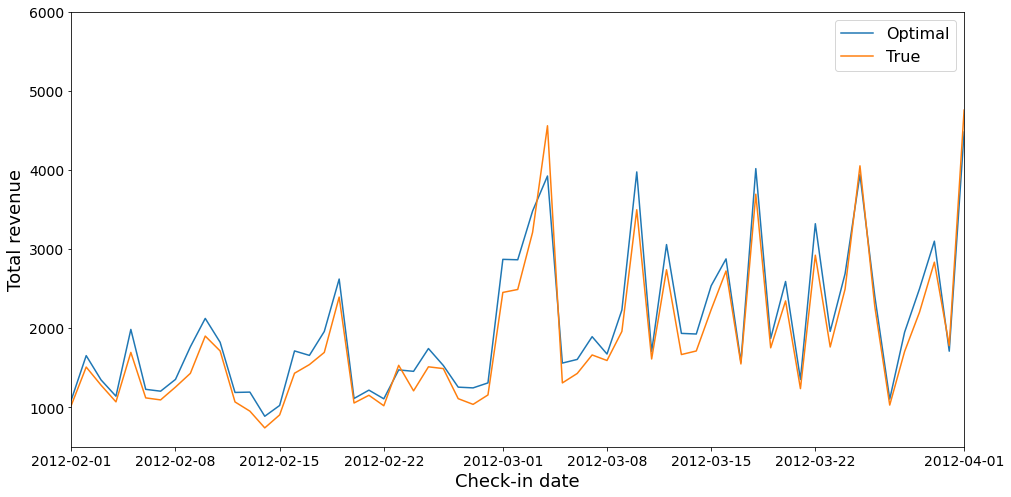Figure 12. Comparison of the results obtained for each check-in day in February and March. From top to bottom, the average daily rate, the number of rooms booked and the total profit obtained for each day. The expected results using the optimal strategy are shown in blue, and the actual values in orange.

Again, the top panel shows that the recommended average price per room is lower to the one actually charged. This discount causes the expected demand to be significantly higher than the actual demand, as observed in the middle panel. Finally, the bottom panel shows that total profits are generally higher using the optimal pricing strategy than those achieved with the current strategy, which proves that the expected increase in demand compensates the lower rates and thus the strategy is optimal.

Finally, we have computed the expected total profit using the proposed strategy, which corresponds to the average obtained over all the Monte Carlo simulations. On the other hand, the actual total profit obtained in the forecast period (year 2012) has been calculated in order to compare the results. Table 1 summarizes the results obtained.

The proposed strategy is expected to increase total benefits by an average of around 7%. Taking into account the standard deviation over all simulations, the expected total benefit is between 6 and 8% above the total profit obtained with the current basic pricing strategy.

## 8. Conclusions and future work

In this article we have presented a demand forecasting model and a dynamic pricing strategy in the hotel context.

First, demand has been modeled as the product of two functions: the total demand size, which depends on the day of check-in, and the shape of demand according to the time in advance. After a previous step of deseasonalization, we have forecasted the demand size series using the Holt-Winters exponential smoothing method. After reintroducing seasonality in the projected series, we have seen that the prediction reasonably approximates the real demand in the forecast period. On the other hand, we have calculated the shape of the demand from the historical data, differentiating by each seasonality period. Using these two functions, we simulated the future demand for each check-in day and booking day using the Monte Carlo method.

On the other hand, the effect of price on demand has been inferred from historical data. In order to obtain a negative elasticity, both variables have been normalized with respect to reference values. Then, normalized demand has been linearly fitted on normalized price for each seasonal period, obtaining negative slopes in all cases.

This information was then used to calculate the optimal price to be set to maximize profits in the study period. To do this, a parametric model for the price has been presented, based on multipliers according to the lead time and the number of rooms available. We have reproduced the inferred effect of the price on the previously simulated reference demand, and in this way we have calculated the expected profits with each choice of multiplier parameters. Using an evolutionary strategy we calculated the best set of parameters, and found that it is indeed expected to improve the results obtained with the current strategy.

Finally, it is worth mentioning that this study can be extended in several directions. To begin with, we could consider a data set with information broken down to a booking. For example, if we have information such as the number of nights or the number of rooms in each reservation, we can infer the distribution of these variables and include them in our simulation. We could also try to include multipliers for these variables in the optimal pricing model, for example based on discounts for long stays or large groups. Of course, different predictions can also be considered for different types of rooms and, if we have a segmentation of customers, the price elasticity of demand for each segment can be studied in a differentiated way.

On the other hand, more complex models could be used for the various problems that have been addressed throughout the paper. For example, algorithms based on recurrent neural networks can be used to predict future demand. Non-linear models can also be considered to estimate the effect of demand on price, taking into account saturation effects for very high and very low prices. Finally, one could add complexity to the multiplier model that has been used for price, for example by adding more multipliers if we have more variables, as discussed above. However, one could consider a completely different parametric model, or even non-parametric models that would require solving an optimization problem in order to find the optimal price for each day.